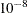# The ICLIFETEST Procedure

### PROC ICLIFETEST Statement

• PROC ICLIFETEST <options>;

The PROC ICLIFETEST statement invokes the ICLIFETEST procedure. Table 62.1 summarizes the options available in the PROC ICLIFETEST statement.

Table 62.1: Options Available in the PROC ICLIFETEST Statement

Option

Description

Input and Output Data Sets

Specifies the input SAS data set

Names an output data set to contain survival estimates and confidence limits

Nonparametric Estimation

Requests that the simple bootstrap method be used for computing standard errors of the survival estimates

Specifies details for multiple imputations used for computing standard errors and estimating covariance matrix of the generalized log-rank statistic

Displays the iteration history of nonparametric estimation

Set the maximum number of iterations allowed for the estimation method

Specifies the method to estimate the survival function

Sets the log-likelihood convergence criterion

Confidence Limits for Survival Function

Sets the level for confidence interval estimation

Specifies the transformation applied to the survival function for computing confidence limits

ODS Graphics

Specifies the maximum time value for plotting

Specifies plots to display

Control Output

Suppresses the display of printed output

Suppresses the display of summary of censored and uncensored values

Displays the Turnbull intervals

Miscellaneous

Sets the level for confidence intervals for survival time quartiles

Treats missing values as a valid stratum or group for the variables specified in the STRATA and TEST statements

Sets the tolerance for testing singularity of the covariance matrix of test statistics

If no options are specified, PROC ICLIFETEST computes and displays a nonparametric estimate of the survival function. If ODS Graphics is enabled, a plot of the estimated survival function is also displayed.

You can specify the following options.

ALPHA=
specifies the levelfor the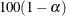% confidence intervals for the survival functions. For example, ALPHA=0.05 requests 95% confidence limits. By default, ALPHA=0.05.

ALPHAQT=specifies the levelfor the% confidence intervals for the quartiles of the survival time. For example, ALPHAQT=0.05 requests a 95% confidence interval. By default, ALPHAQT=0.05.

BOOTSTRAP <(options)>
BOOT <(options)>

performs simple bootstrap sampling and computes bootstrap standard errors of nonparametric survival estimates. You can specify the following suboptions to control how the bootstrap samples are generated:

NBOOT

specifies the number of bootstrap samples to be generated. The default value is 1,000.

SEED

specifies the seed to generate bootstrap samples. The default seed is selected randomly.

CONFTYPE=ASINSQRT | LINEAR | LOG | LOGLOG

specifies the transformation to be applied to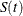to obtain pointwise confidence intervals for the survival function in addition to the confidence intervals for the quartiles of the survival times. You can specify the following keywords:

ASINSQRT

specifies the arcsine–square root transformation,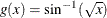LOGLOG

specifies the log–log transformation,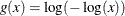This is also referred to as the log cumulative hazard transformation, because it applies the log transformation to the cumulative hazard function. Collett (1994) and Lachin (2000) call it the complementary log-log transformation.

LINEAR

specifies the identity transformation,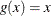LOG

specifies the log transformation,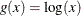LOGIT

specifies the logit transformation,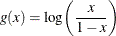By default, CONFTYPE=LOGLOG.

DATA=SAS-data-set

names the SAS data set that PROC ICLIFETEST reads. By default, the most recently created SAS data set is used.

IMPUTE <(options)>
IM <(options)>

specifies details for multiple imputations. You can specify the following two suboptions to control how the samples are generated:

NIMSE

specifies the number of imputation samples to be generated for computing standard errors of the survival estimates. The default value is 1,000.

NIMTEST

specifies the number of imputation samples to be generated for estimating the covariance matrix of the generalized log-rank statistic. The default value is 1,000.

SEED

specifies the seed to generate imputation data sets. The default seed is selected randomly.

ITHISTORY

prints the iteration history of the nonparametric estimation, including the log likelihood, the probability estimate that is associated with each Turnbull interval, and whether the EM or ICM method is used for each iteration.

MAXITER=n
MAXIT=n

specifies the maximum number of iterations for estimating the survival function. The default value depends on the estimation method as follows:

• EMICM method: 200

• ICM method: 200

• Turnbull method: 500

Note that you specify the method with the METHOD= option.

MAXTIME=value

specifies the maximum value of the time variable that is allowed in plots so that outlying points do not determine the scale of the time axis of the plots. This option affects only the way in which plots are displayed and has no effect on any calculations.

METHOD=TURNBULL | ICM | EMICM

specifies the method to be used for computing survival function estimates. You can specify the following:

TURNBULL
EM

requests that the Turnbull method be used to estimate the survival function.

EMICM

requests that the EMICM algorithm be used to estimate the survival function.

ICM

requests that the iterative convex minorant algorithm be used to estimate the survival function.

By default, METHOD=EMICM.

MISSING

allows missing values to be a stratum level or a valid group for the variables that are specified in the STRATA and TEST statements.

NOPRINT

suppresses the display of output. This option is useful when only an output data set is needed. It temporarily disables the Output Delivery System (ODS); for more information about ODS, see Chapter 20: Using the Output Delivery System.

NOSUMMARY

suppresses the summary table of the number of censored and uncensored values.

OUTSURV=SAS-data-set
OUTS=SAS-data-set

creates an output SAS data set to contain the estimates of the survival function and corresponding confidence limits for all the strata. For more information about the contents of the OUTSURV= data set, see the section OUTSURV= Data Set.

PLOTS<(global-plot-options)>=plot-request <(options)>
PLOTS<(global-plot-options)>=(plot-request <(options)> <…plot-request <(options)>>)

controls the plots that are produced by using ODS Graphics. When you specify only one plot-request, you can omit the parentheses around it. Here are some examples:

plots=none
plots=(survival(cl) logsurv)
plots(only)=hazard


ODS Graphics must be enabled before plots can be requested. For example:

ods graphics on;

proc iclifetest plots=survival;
time (Left,Right);
run;

ods graphics off;


For more information about enabling and disabling ODS Graphics, see the section Enabling and Disabling ODS Graphics in Chapter 21: Statistical Graphics Using ODS.

If ODS Graphics is enabled but you do not specify the PLOTS= option, then by default PROC ICLIFETEST produces a plot of the estimated survival functions.

You can specify the following global-plot-option:

ONLY

specifies that only the specified plots in the list be produced; otherwise, the default survival function plot is also displayed.

You can specify the following plot-requests and their options:

ALL

produces all appropriate plots. Specifying PLOTS=ALL is equivalent to specifying PLOTS=(SURVIVAL LOGSURV LOGLOGS HAZARD).

HAZARD <(hazard-options)>
H <hazard-options>

plots the kernel-smoothed hazard functions. You can specify the following hazard-options.

BANDWIDTH=value | numeric-list | RANGE(lower,upper)
BW=

specifies the bandwidth for kernel-smoothed estimation of the hazard function. You can specify one of the following:

value

sets the bandwidth to the given value.

numeric-list

selects the bandwidth from the specified numeric-list that minimizes the cross validation pseudo-likelihood.

RANGE(lower,upper)

selects from the interval (lower, upper) the bandwidth that minimizes a cross validation pseudo-likelihood function. PROC ICLIFETEST uses the golden section search algorithm to find the minimum. If the interval contains more than one local minimum, there is no guarantee that the local minimum that the algorithm finds is also the global minimum.

For more information about the cross validation pseudo-likelihood function, see the section Kernel-Smoothed Estimation. By default, BANDWIDTH= RANGE(0.1b,0.25b), where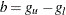;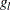is the left boundary of the first Turnbull interval; and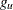is the right boundary of the last Turnbull interval if it is finite or the left boundary of that interval multiplied by 1.1 otherwise.

SAMPLING=LEAVEONE | RANDOM

specifies how to partition the data set to form cross validation groups. You can specify the following values:

LEAVEONE

partitions the data set into leave-one-out subsets.

RANDOM

randomly assigns observations to cross validation groups with equal probabilities.

By default, SAMPLING=RANDOM.

GRIDL=number

specifies the lower grid limit for the kernel-smoothed estimate. The default value is the time origin.

GRIDU=number

specifies the upper grid limit for the kernel-smoothed estimate. The default value is the maximum input boundary value.

KERNEL=kernel-option

specifies the kernel to be used. You can specify the following values:

BIWEIGHT
BW

uses the following kernel: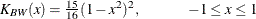EPANECHNIKOV
E

uses the following kernel: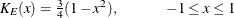UNIFORM
U

uses the following kernel: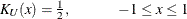By default, KERNEL=EPANECHNIKOV.

CVFOLD=number

specifies the number of cross validation groups. This option is applicable only when SAMPLING=RANDOM. The default number is either 5 or the sample size, whichever value is less.

CVGRID=number

specifies the number of grid points to use in determining the cross validation pseudo-likelihood. By default, CVGRID=51.

HGRID=number

specifies the number of grid points for discretizing the smoothed hazard function. By default, HGRID=101.

LOGLOGS
LLS

plots the log of the negative log of the estimated survival function versus the log of time.

LOGSURV
LS

plots the negative log of the estimated survival function versus time.

NONE

suppresses all plots.

SURVIVAL <(CL | FAILURE | NODASH | STRATA | TEST)>
S <(CL | FAILURE | STRATA | TEST)>

plots the estimated survival function. You can customize the display by specifying the following values:

CL

displays pointwise confidence limits for the survival function.

FAILURE
F

changes all the displays for survival function to those for the failure function. For example, if you specify both the FAILURE and CL options, the plot displays the failure curves in addition to pointwise confidence limits for the failure function.

NODASH

suppresses the plotting of dashed lines as linear interpolations for the Turnbull intervals.

STRATA=INDIVIDUAL | OVERLAY | PANEL

specifies how to display the survival or failure curves for multiple strata. This option has no effect if there is only one stratum. You can specify one of the following values:

INDIVIDUAL
UNPACK

requests that a separate plot be displayed for each stratum.

OVERLAY

requests that the survival or failure curves for the strata be overlaid on the same plot.

PANEL

requests that separate plots for the strata be organized into panels of two or four plots, depending on the number of strata.

By default, STRATA=OVERLAY.

TEST

displays the p-value for a K-sample test that is specified in the TEST statement.

SHOWTI

presents the survival estimates in terms of Turnbull intervals.

SINGULAR=value

sets the tolerance for testing the singularity of the covariance matrix of test statistics.

TOLLIKE=value

sets the log-likelihood convergence criterion for the estimation algorithm. The default value depends on the estimation method as follows:

• EMICM method: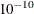• ICM method:• Turnbull method: